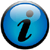# Processing input from one or more files

Perl allows very fine control about how to process and combine input from multiple files. Unfortunately, the code becomes a bit more complexes, pushing it to the limit of one-liners.
1. Add columns with minimum and maximum value for each row in a tab-delimited text file:
```perl -MRegexp::Common -F"\t" -lane '@vals = (); foreach (@F) { push @vals, \$_ if (/^\$RE{num}{real}\$/)} @vals = (sort { \$a <=> \$b } @vals); print "\$_\t\$vals\t\$vals[-1]"' input > output

```
2. Report frequency of elements in the third column (index 2) of a tab-delimited text file:
```perl -F"\t" -lane '\$freq{\$F}++; END {foreach (sort keys %freq) {print "\$_ -> \$freq{\$_}";}}' input

```
3. Print the different flags set in a BAM file and how many entries are associated with it. This could be used to check if there is an even number of reads mapping to both strands:
```samtools view bam_file | perl -lne '@h = split "\t", \$_; \$f{\$h}++; END { foreach (sort keys %f) { print "\$_\t\$f{\$_}";}}'

```
4. Read files with gene IDs and report in decreasing order in how many files each ID is found:
```perl -e 'foreach (@ARGV) {open (IN, \$_); while (<IN>) {chomp; \$in{\$_}++;}} foreach (sort { \$in{\$a} <=> \$in{\$b} } keys %in) { print "\$_ -> \$in{\$_}\n";}' file*

```
5. Print the reverse complement of all sequences in a fasta file:
```perl -lne 'if (/>/) {\$h = \$_} else {\$in{\$h} .= \$_;} END { foreach (sort keys %in) { \$s = lc reverse \$in{\$_}; \$s =~ tr/acgt/tgca/; print "\$_\n\$s"}}' input > output

```
6. Extract a sub-sequence (50 basepairs at position 1000) from a file with a single fasta sequence:
```perl -lne 'next if (/^>/); \$s .= \$_; END { print(substr \$s, 1000-1, 50) }' input
try it out with an example input file
```
7. Report input lines that differ in their first element between two files:
```perl -e '\$f1 = shift; open (IN, \$f1); while (<IN>) {@h = split; \$f1{\$h}++;} close IN; \$f2 = shift; open (IN, \$f2); while (<IN>) {@h = split; \$f1{\$h}--;} foreach (sort keys %f1) { print "\$_ -> \$f1{\$_}\n" if (\$f1{\$_})}' file1 file2 > diff.txt
Lines only found in the first file will be printed with a value of 1, lines only in the second with a value of -1.

```
8. Use a file of IDs to filter lines from another file and report IDs that were not found:
```perl -e '\$f = shift; open (IN, \$f); while (<IN>) {@h = split; \$f{\$h}++;} close IN; \$f = shift; open (IN, \$f); while (<IN>) {chomp; @h = split; if (defined \$f{\$h}) {print "\$_\n"; \$f{\$h} = 0}} foreach (sort keys %f) {print STDERR "not found: \$_\n" if (\$f{\$_})}' filter_file input > output
```9. Combine two files using IDs from first column as key:
```perl -e 'foreach \$f (@ARGV) {open (IN, \$f); while (<IN>) {chomp; @h = split /\t/, \$_; \$in{\$h}{\$f} = \$_; } close IN; } foreach (sort keys %in) { print "\$in{\$_}{\$ARGV}\t\$in{\$_}{\$ARGV}\n";}' input1 input2 > combined
```10. Print all palindromes of length 15 found in a sequence file using a sliding window approach:
```perl -lne '\$in .= \$_; END {foreach (0..length(\$in)-15) { \$t = substr \$in, \$_, 15; print "\$i_: \$t" if (\$t eq reverse(\$t)) } }' input
```